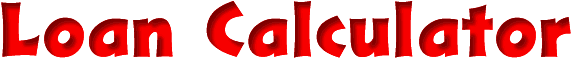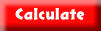This page will calculate the approximate monthly payments and the total interest you will pay for a loan. It's approximate since it makes its calculations using interest that's compounded monthly and many consumer loans for items like cars are compounded daily. The payment amounts calculated for these types of loans will be slightly low.

The inputs are the amount borrowed, the interest rate, and either the number of monthly payments or the exact monthly payment you want to make. If you specify the number of months to pay, the payment amount and total interest are calculated. If you set the number of months to zero or clear the field, and specify the amount you want to pay in the monthly payment field, the number of monthly payments required and a possible different last payment amount will fill in.

The defaults are for a \$10,000.00 loan at an annual interest rate of 8.25% over a period of 48 months. If you hit the clear button more then once the form will toggle back and forth between cleared fields and these initial defaults. Remember the results are approximate.

 Amount to borrow: (digits and decimal only, ie. 10000.00) dollars Annual interest rate: (digits and decimal only, ie. 8.25) percent Number of monthly payments: (digits only, ie. 48) months Monthly payment amount: (digits and decimal only, ie. 250.00) dollars Last payment if different: dollars Total interest paid: dollarsNOTE: This form uses JavaScript which won't work with some older browsers. Sorry.